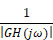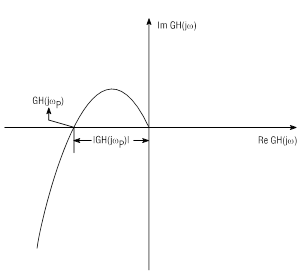### Relative stability

The most common relative stability measures are the gain margin, gain crossover frequency, phase margin, and phase crossover frequency. These quantities can be deduced from the Nyquist stability plot.

The phase crossover frequency is the frequency at which the phase angle of GH(jω) is -180o. This is the frequency at which the Nyquist plot crosses the negative real axis. The gain margin is equal to:These quantities are shown in the following figure:The gain crossover frequency is the frequency at which |GH(jω)| = 1. The phase margin is the angle by which the Nyquist plot must be rotated to intersect (pass through) the (-1,0) point. These quantities are depicted in the figure below.